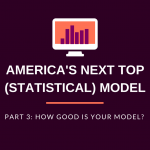## Rootscamp’s Next Top Model–How Good is Your Model?# Rootscamp’s Next Top Model–How Good is Your Model?

Quick Tip

This post is the final part of a three-part series based on a training given at NOI’s RootsCamp Conference in December.  Read part one to learn what statistical models are, and read part two to learn how to build a model.

We often build statistical models because we want to use them to inform our decisions. This could mean choosing who should be included in a GOTV plan or deciding who should receive a fundraising email.  When using models to make these decisions, it is generally useful to have an idea of how accurate our model’s predictions are.

There are many ways to measure the accuracy of a model.  Here we will discuss three of these methods–going from more basic to more sophisticated.

Method 1: Misclassification Rate

The first method tells us how often our predictions fail to match up with the data.  To see how this works, we’ll use an example from the previous post where we attempted to model how people in a particular district would vote based on their ages.

To measure the misclassification rate of our model, we can divide the chart into four quadrants.  The vertical line on the chart below corresponds to 48 years–the age where our model says there is an exactly 50-50 chance that a randomly selected person will vote for the Republican.

According to our model, anyone who is older than 48 (to the right of the line) has more than a 50% chance of voting Republican.  This means that our best guess for anyone over 48 is that they will vote Republican, and our best guess for everyone under 48 is that they will vote Democrat.  By dividing the chart into four quadrants, we can easily see which predictions are right (correctly classified) and which are wrong (misclassified).

Unfortunately, there are several problems with simply using the misclassification rate to judge a model.  We will focus on just one of these problems.  In the previous post in the series, we discussed that the data on which we build statistical models often comes from some sort of survey or poll.  What if political preferences are not really correlated with age, but, by chance, we happened to survey lots of old Republicans and young Democrats?  Our model would tell us that older people are people are more likely to vote Republican, but this wouldn’t really be true in the general population.

Using misclassification to evaluate our model tells us how well the model fits our sample, but it does not allow us to estimate how well our model fits the population as a whole.

Method 2: Using a Test Set

One way to partially solve the problem described above is to use what’s called a test set.  To do this, we randomly divide our sample into two groups: one group is called the training set and the other is called the test set.

For this process, we’re testing whether our model still fits when we apply it to a different set of data. We do this by separating the data into two sets. One set is called the “training set” and the other set is called the “test set.” We create our model based off of only the data in the training set. Then we test the model on–you guessed it–the test set. We test it by calculating the misclassification rate (the same way we described it in the section above) for our test set. This helps us to know if whether we built a model that only fit that one particular set of data or if we built a model that fits a general trend.

Unfortunately, there is still a problem with this method.  Consider the situation where the model says that there is a 51% chance that a particular person will vote Republican but that person actually votes for the Democrat.  Technically, the model was wrong, but this mistake isn’t as bad as if the model said there was a 99% chance of this person voting Republican.  If we use this method to judge a model, we are not distinguishing between these two types of situations.

Method 3: Cross-Validation

A slight adjustment to Method 2 creates a 3-step technique that works much better:

1. First, use Method 2 to calculate the misclassification rate on the test set.
2. Then, switch the two sets–build the model on what was the test set, and measure the misclassification rate on what was the training set.
3. Finally, calculate the average of the misclassification rates from steps 1 and 2.

This method can be extended by dividing the data into more than two groups.  For example, if we divide the data into three groups:

1. Combine groups 1 and 2 to create a training set.  Test this model on group 3.
2. Combine groups 1 and 3 to create a training set.  Test this model on group 2.
3. Combine groups 2 and 3 to create a training set.  Test this model on group 1.

Instead of only three groups, we can divide our data into as many groups as we want (up to the point where there is just one person in each group).  However, it has been found that this technique generally works best when the data is divided into five to ten groups.

Using cross-validation, we not only can build models to predict who will vote in a given election or who might donate to our campaign, but we can also get an idea of how accurate our predictions are.

What’s Next?

These posts have just scratched the surface of model-building, but if you’re interested in learning more, there are a lot of great free resources online:

## Written By